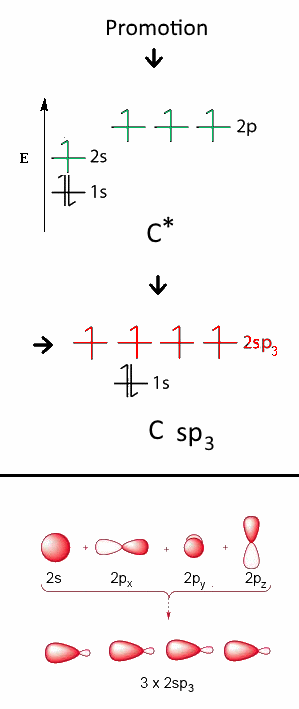# The hybridization of orbitals

## Electronic structure of the isolated carbon atom

$1s^2\; 2s^2\;2p_x^1\;2p_y^1$ is the structure of isolated C determined by the quantum theory.This structure would imply a geometry with angles different from those actually observed!

## Electronic structure of the carbon atom in the organic moleculesLinus Pauling 1901 - 1994

Linus Pauling, reasoning on quantum theory, found that, depending on its environment, the $C$ atom orbitals could result as a "superposition" of atomic orbitals. By analogy with biology, these "mixtures" are called hybridized orbitals. Naively, the phenomenon is described by first admitting electronic excitation, "promotion":First, an electron is uplifted from the $2s$ orbital to the free $2p_z$ orbital. After that, the atom is ready for the following hybridizations:

### The $sp_3$ hybridizationThis hybridization is a "mixture" of $2s\;2p_x\;2p_y$ and $2p_z$ to form four new orbitals with intermediary energy and designated by $2sp_3$ each. These new orbitals have completely equivalent structures and each contains one electron. The C atom has now a tetrahedral structure:Example: Methane

Methane has a tetrahedral structure with 4 $\sigma$ bonds realised by head-on overlapping $2sp_3\; -\; 1s$### The $sp_2$ hybridizationThis hybridization is a "mixture" of $2s\;2p_x$ and $2p_y$ to form three new orbitals with intermediary energy and designated by $2sp_2$ each. These new orbitals have completely equivalent structures and each contains one electron. The $C$ atom has now a planar triangular structure, the orbital $2p_z$ remains intact:Example: Ethene

Ethene (ethylene) has a planar sructure - with 4 $\sigma$ $C-H$ bonds realised by head-on overlapping $2sp_2\; -\;1s$, - one $\sigma$ $C-C$ bond realised by head-on overlapping $2sp_2\; - \;2sp_2$Backbone of ethene (without $\pi$ bond ) with 1 ( one single !) $\pi$ $C-C$ bond realised by lateral overlapping $2p_z\; -\;2p_z$ of the two lobes of $2p_z$ orbitals on the adjacent carbon atoms.Complete structure of ethene (with the $\pi$ bond)

Special case: BenzeneBenzene is constituted by a regular hexagonal ring formed of 6 carbon atoms hybridized $sp_2$ As in the case of ethene, $C-C$ bonds are $\sigma$ bonds $(2sp_2\;-\;2sp_2)$, $C-H$ bonds are $\sigma$ bonds $(2sp_2\;-\;1s)$ However, the six $2p_z$ electron orbitals coalesce here in an electronic cloud, called a $\pi$ cloud forming two regular toroids above and below the ring.

### The $sp$ hybridizationThis hybridization is a "mixture" of $2s$ and $2p_x$ to form two new orbitals of intermediate energy and designated $2sp$ each. These new orbitals have completely equivalent structures and each contains one electron. The $C$ atom has a linear structure, orbitals $2p_y$ and $2p_z$ remaining intact:Example: Acetylene (ethyne)

Acetylene (ethyne) has a linear structure - with 2 $\sigma$ $C-H$ bonds realised by head-on overlapping $2sp\; - \;1s$, - one $\sigma$ $C-C$ bond realised by head-on overlapping $2sp\;- \;2sp$Backbone of ethyne (without the $\pi$ bonds) - with 2 (only two !) $\pi$ $C-C$ bonds by lateral overlapping $2p_y\; - \;2p_y$ and $2p_z\; - \;2p_z$Complete structure of ethyne (with the two $\pi$ bonds)